Approximate Nonlinear Behavior Using Array of LTI Systems

This example shows how to approximate the nonlinear behavior of a system as an array of interconnected LTI models.

The example describes linear approximation of pitch axis dynamics of an airframe over a range of operating conditions. The resulting array of linear systems is used to create a linear parameter varying (LPV) representation of the dynamics. The LPV model serves as an approximation of the nonlinear pitch dynamics.

Linear Parameter Varying Models

In many situations you must approximate the nonlinear dynamics of a system using simpler linear systems. A single linear system provides a reasonable model for behavior limited to a small neighborhood around an operating point of the nonlinear system. When you must approximate the nonlinear behavior needs over a range of operating conditions, you can use an array of linear models that are interconnected by suitable interpolation rules. Such a model is called an LPV model.

To generate an LPV model, the nonlinear model is trimmed and linearized over a grid of operating points. For this purpose, the operating space is parameterized by a small number of scheduling parameters. These parameters are often a subset of the inputs, states, and output variables of the nonlinear system. Important considerations in the creation of LPV models are the identification of a scheduling parameter set and the selection of a range of parameter values at which to linearize the model.

To illustrate this approach, this example approximates the pitch dynamics of an airframe.

Airframe Pitch Dynamics

Consider a three-degree-of-freedom model of the pitch axis dynamics of an airframe. The states are the Earth coordinates, the body coordinates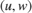, the pitch angle, and the pitch rate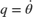. The following figure summarizes the relationship between the inertial and body frames, the flight path angle, the incidence angle, and the pitch angle.The airframe dynamics are nonlinear and the aerodynamic forces and moments depend on speedand incidence. The scdairframeTRIM model scdairframeTRIM describes these dynamics.

open_system('scdairframeTRIM')Batch Linearization Across the Flight Envelope

Use the speedand the incidence angleas scheduling parameters; that is, trim the airframe model over a grid ofandvalues. These values are two of the five outputs of the scdairframeTRIM model.

Assume that the incidencevaries between -20 and 20 degrees and that the speedvaries between 700 and 1400 m/s. Use a 15-by-12 grid of linearly spaced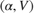pairs for scheduling.

nA = 15;   % number of alpha values
nV = 12;   % number of V values
alphaRange = linspace(-20,20,nA)*pi/180;
VRange = linspace(700,1400,nV);
[alpha,V] = ndgrid(alphaRange,VRange);

For each flight condition, linearize the airframe dynamics at trim (zero normal acceleration and pitching moment). Doing so requires computing the elevator deflection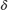and pitch ratethat result in steadyand.

For each flight condition, you:

• Specify the trim condition using the operspec function.

• Compute the trim values ofandusing the findop function.

• Linearize the airframe dynamics for the resulting operating point using the linearize function.

The body coordinates,, are known states for trimming. Therefore, you must provide appropriate values for them, which you can specify explicitly. However, in this example, let the model derive these known values based on eachpair. For each flight condition, update the values in the model and create an operating point specification. Repeat these steps for all 180 flight conditions.

clear op report
for ct = 1:nA*nV
alpha_ini = alpha(ct);      % Incidence [rad]
v_ini = V(ct);              % Speed [m/s]

% Specify trim condition
opspec(ct) = operspec('scdairframeTRIM');

opspec(ct).States(1).Known = [1;1];

opspec(ct).States(3).Known = [1 1];

opspec(ct).States(2).Known = 1;

opspec(ct).States(4).Known = 0;

end
opspec = reshape(opspec,[nA nV]);

Trim the model for all of the operating point specifications.

opt = findopOptions('DisplayReport','off', 'OptimizerType','lsqnonlin');
opt.OptimizationOptions.Algorithm = 'trust-region-reflective';
[op,report] = findop('scdairframeTRIM',opspec,opt);

The op array contains the operating points found by findop that will be used for linearization. The report array contains a record of input, output, and state values at each point.

To linearize the model, first specify linearization input and output points.

io = [linio('scdairframeTRIM/delta',1,'in');            % delta
linio('scdairframeTRIM/Airframe Model',1,'out');  % alpha
linio('scdairframeTRIM/Airframe Model',2,'out');  % V
linio('scdairframeTRIM/Airframe Model',3,'out');  % q
linio('scdairframeTRIM/Airframe Model',4,'out');  % az
linio('scdairframeTRIM/Airframe Model',5,'out')]; % gamma

Linearize the model for each of the trim conditions. Store linearization offset information in the info structure.

linOpt = linearizeOptions('StoreOffsets',true);
[G,~,info] = linearize('scdairframeTRIM',op,io,linOpt);
G = reshape(G,[nA nV]);
G.u = 'delta';
G.y = {'alpha','V','q','az','gamma'};
G.SamplingGrid = struct('alpha',alpha,'V',V);

G is a 15-by-12 array of linearized plant models at the 180 flight conditions. The plant dynamics vary substantially across the flight envelope, including scheduling locations where the local dynamics are unstable.

bodemag(G(3:5,:,:,:))
title('Variations in airframe dynamics')LPV System Block

The LPV System block facilitates simulation of linear parameter varying systems. The block requires the state-space system array G that you generated using batch linearization. You also augment this information with the input, output, state, and state derivative offsets from the info structure.

Extract the offset information.

offsets = getOffsetsForLPV(info);
xOffset = offsets.x;
yOffset = offsets.y;
uOffset = offsets.u;
dxOffset = offsets.dx;

LPV Model Simulation

The scdairframeLPV model, which contains an LPV System block that uses the linear system array G and the corresponding offsets.

This model uses an input signal based on a desired trajectory of the airframe. This signal u and corresponding time vector t are saved in the scdairframeLPVsimdata.mat file, which is loaded by the model. Specify the initial conditions for simulation.

alpha_ini = 0;
v_ini = 700;
x0 = [0; 700; 0; 0];

Open and simulate the model.

open_system('scdairframeLPV')
sim('scdairframeLPV')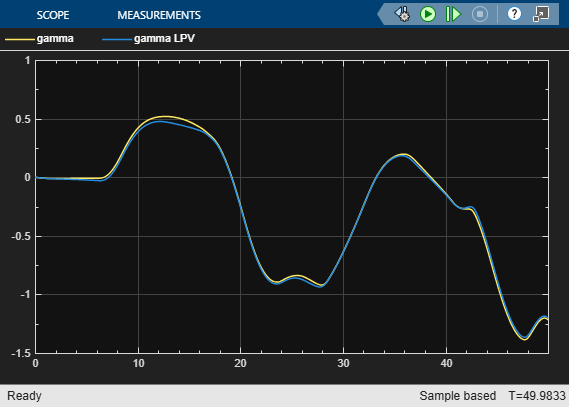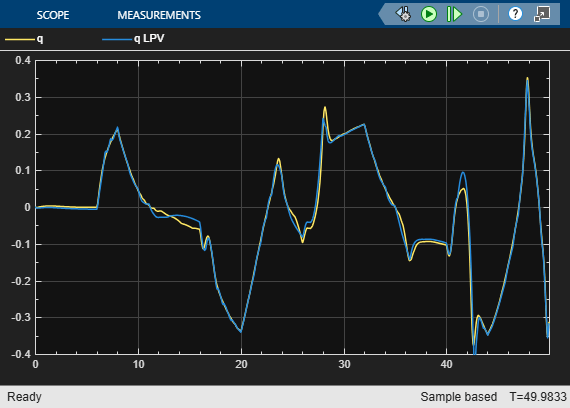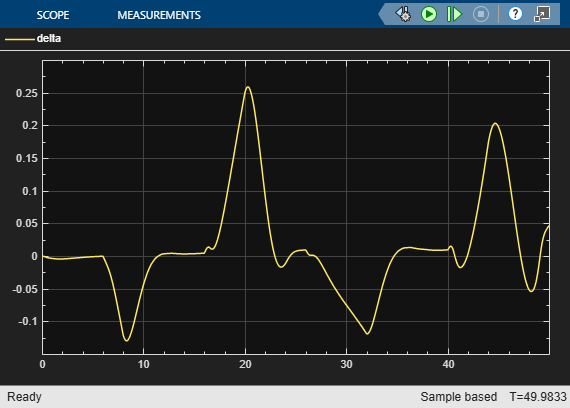The simulation shows good emulation of the airframe response by the LPV system. For this simulation you specified a fine gridding of the scheduling space leading to a large number (180) of linear models. Large array sizes can increase implementation costs. However, the advantage of LPV representations is that you can adjust the scheduling grid, and hence the number of linear systems in the array, based on:

• The scheduling subspace spanned by the anticipated trajectory

• The level of accuracy desired in an application

The former information helps reduce the range for the scheduling variables. The latter helps pick an optimal resolution (spacing) of samples in the scheduling space.

Plot the actual trajectory of scheduling variables in the previous simulation against the backdrop of the gridded scheduling space. Theoutputs were logged using their corresponding scopes (contained inside the Compare Responses block of scdairframeLPV).

Stable = false(nA,nV);
for ct = 1:nA*nV
Stable(ct ) = isstable(G(:,:,ct));
end
alpha_trajectory = Alpha_V_Data.signals(1).values(:,1);
V_trajectory = Alpha_V_Data.signals(2).values(:,1);

plot(alpha(Stable)*180/pi,V(Stable),'g.',...
alpha(~Stable)*180/pi,V(~Stable),'k.',...
alpha_trajectory,V_trajectory,'r.')
title('Trajectory of scheduling variables')
xlabel('\alpha')
ylabel('V')
legend('Stable locations','Unstable locations','Actual trajectory')The trajectory traced during simulation is shown in red. It traverses both the stable and unstable regions of the scheduling space.

Suppose that you want to implement this model on target hardware for input profiles similar to the one used for the previous simulation, while using the least amount of memory. The simulation suggests that the trajectory stays mainly in the 890 to 1200 m/s range of velocities and -15 to 12 degree range of incidence angle. Find the indices in the scheduling space that correspond to this operating region.

I1 = find(alphaRange>=-15*pi/180 & alphaRange<=12*pi/180);
I2 = find(VRange>=890 & VRange<=1200);

To reduce the number of flight conditions, you can also increase the spacing between the sampling points. For example, extract the indices for every third sample along thedimension and every second sample along thedimension.

I1 = I1(1:2:end);
I2 = I2(1:3:end);

Extract the subset of the LTI system array.

Gr = G(:,:,I1,I2);
size(Gr)
5x2 array of state-space models.
Each model has 5 outputs, 1 inputs, and 4 states.

The new sampling grid, Gr, has a more economical size of 5-by-2. Simulate the reduced model and check its fidelity in reproducing the original behavior.

Configure the LPV System block to use the reduced model and corresponding offsets.

lpvblk = 'scdairframeLPV/LPV System';
set_param(lpvblk,...
'sys','Gr',...
'uOffset','uOffset(:,:,I1,I2)',...
'yOffset','yOffset(:,:,I1,I2)',...
'xOffset','xOffset(:,:,I1,I2)',...
'dxOffset','dxOffset(:,:,I1,I2)')
sim('scdairframeLPV')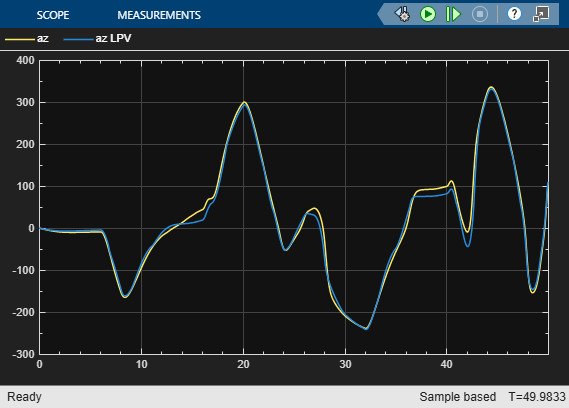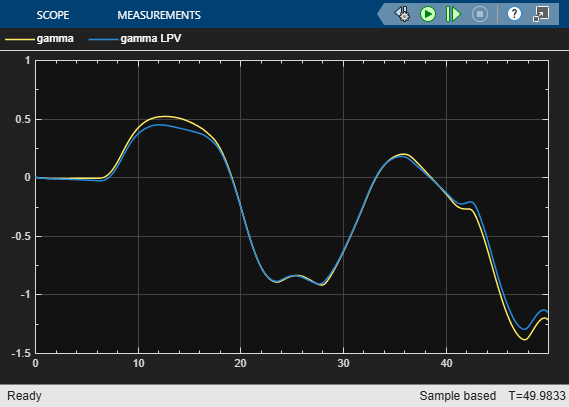There is no significant reduction in overlap between the response of the original model and its LPV proxy.

The LPV model can serve as a proxy for the original system in situations where faster simulations are required. The linear systems used by the LPV model can also be obtained using system identification techniques (with additional care required to maintain state consistency across the array). The LPV model can provide a good surrogate for initializing Simulink® Design Optimization™ problems and performing fast hardware-in-loop simulations.

bdclose('scdairframeLPV')
bdclose('scdairframeTRIM')